# The single facility location problem with time-dependent weights and relocation cost over a continuous time horizon

## Abstract

In this study, we investigate the problem of locating a facility in continuous space when the weight of each existing facility is a known linear function of time. The location of the new facility can be changed once over a continuous finite time horizon. Rectilinear distance and time- and location-dependent relocation costs are considered. The objective is to determine the optimal relocation time and locations of the new facility before and after relocation to minimize the total location and relocation costs. We also propose an exact algorithm to solve the problem in a polynomial time according to our computational results.

This is a preview of subscription content, access via your institution.

## References

• Bastian M and Volkmer M (1992). A perfect forward procedure for a single facility dynamic location/relocation problem. Operations Research Letters 12 (1): 11–16.

• Bose S, Goswami A and Chaudhuri KS (1995). An EOQ model for deteriorating items with linear time-dependent demand rate and shortages under inflation and time discounting. Journal of the Operational Research Society 46 (6): 771–782.

• Brotcorne L, Laporte G and Semet F (2003). Ambulance location and relocation models. European Journal of Operational Research 147 (3): 451–463.

• Domschke W and Drexel A (1985). Location and layout planning: An international bibliography. Lecture Notes in Economics and Mathematical Systems, Vol. 238. Springer: Berlin.

• Drezner Z (1995). Dynamic facility location: The progressive p-median problem. Location Science 3 (1): 1–7.

• Drezner Z and Wesolowsky GO (1991). Facility location when demand is time dependent. Naval Research Logistics 38 (5): 763–777.

• Erkut E, Ingolfsson A and Erdoğan G (2008). Ambulance location for maximum survival. Naval Research Logistics 55 (1): 42–58.

• Farahani RZ and Hekmatfar M (eds). (2009). Facility Location: Concepts, Models, Algorithms and Case Studies. Physica-Verlag Heidelberg: Germany.

• Farahani RZ, Drezner Z and Asgari N (2009). Single facility location and relocation problem with time dependent weights and discrete planning horizon. Annals of Operations Research 167 (1): 353–368.

• Francis RL, McGinnis LF and White JA (1992). Facility Layout and Location: An Analytical Approach, 2nd edn. Prentice Hall: Englewood Cliffs.

• Hamacher HW and Nickel S (1998). Classification of location models. Location Science 6 (1–4): 229–242.

• Linnenluecke MK, Stathakis A and Griffiths A (2011). Firm relocation as adaptive response to climate change and weather extremes. Global Environmental Change 21 (1): 123–133.

• Min H and Melachrinoudis E (1999). The relocation of a hybrid manufacturing/distribution facility from supply chain perspectives: A case study. Omega 27 (1): 75–85.

• Owen SH and Daskin MS (1998). Strategic facility location: A review. European Journal of Operational Research 111 (3): 423–447.

• Schmid V and Doerner KF (2010). Ambulance location and relocation problems with time-dependent travel times. European Journal of Operational Research 207 (3): 1293–1303.

• Scott AJ (1971). Dynamic location-allocation systems: Some basic planning strategies. Environment and Planning 3 (1): 73–82.

• Scypinski S, Sadlowski T and Baiano J (1997). Relocating and reestablishing a robotics laboratory. Laboratory Robotics and Automation 9 (5): 229–236.

• Thanh PN, Bostel N and Péton O (2008). A dynamic model for facility location in the design of complex supply chains. International Journal of Production Economics 113 (2): 678–693.

• Wesolowsky GO (1973). Dynamic facility location. Management Science 19 (11): 1241–1248.

• Wesolowsky GO and Truscott WG (1976). The multi-period location-allocation problem with relocation of facilities. Management Science 22 (1): 57–65.

• Wildstrom DJ (2008). Cost thresholds for dynamic resource location. Discrete Applied Mathematics 156 (10): 1846–1855.

## Acknowledgements

We are grateful for the constructive comments of the associate editor and two reviewers. The second author acknowledges that his research was supported by a grant from the National Natural Science Foundation of China (71271183) and two grants (201011159026, 201111159056) from the University Research Committee of the University of Hong Kong.

## Author information

Authors

### Corresponding author

Correspondence to W Y Szeto.

## Appendices

### The algorithm by Drezner and Wesolowsky (1991)

Step 1::

Calculate X (1)=(x (1), y (1)) and X (2)=(x (2), y (2)) when B=0. Set B=0, K *=K(0, X (1), X (2)) and B *=0.

Step 2::

Calculate the eight break points B n ′ for moving the location of the new facility to its adjacent location (note that there are two break points for each movement) by B n =2(∑ i=1 j u i −∑ i=j+1 m u i )/(∑ i=j+1 m v i −∑ i=1 j v i ), (for moving from j to j+1 or vice versa), for n=1, …, 8.

Step 3::

Find the minimum of these break points B″=min n=1, …, 8{B n |B n >B}. If B″>T or B″ is not defined, set B″=T. The next segment is [B, B″] Find B min by B min =−∑ i=1 m[u i (d i (1)d i (2))]/∑ i=1 m[v i (d i (1)d i (2))] where d i (1)=∣x (1)x i ∣+∣y (1)y i ∣ and d i (2)=∣x (2)x i ∣+∣y (2)y i ∣. If BB min B″ and the denominator of above relation for B min is positive, set B 0=B min ; otherwise, set B 0=B″. Calculate K 0=K(B 0).

Step 4::

If K 0<K *, update K *=K 0 and B *=B 0.

Step 5::

If B″=T, then B * is the optimal solution and K * is the optimal objective value. Otherwise, go to Step 6.

Step 6::

Change the value of x (1), y (1), x (2) or y (2) to its new value based on the value of n for which B″ is obtained in Step 3. Calculate the next B n for this particular n. Set B=B″ and go to Step 3.

### Appendix B

Proof of Lemma 2 Let A *=(B *, X (1)*, X (2)*)=(B *, x (1)*, y (1)*, x (2)*, y (2)*) and W i (1)=∫0 B* w i (t)dt, W i (2)=∫ B* T w i (t)dt; i=1, …, m.

Therefore, we have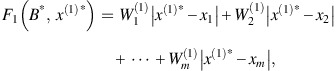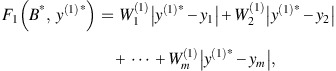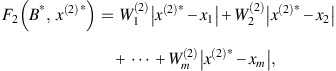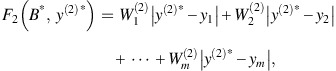and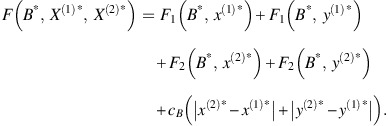Now, we aim to prove that (8) and (9) will hold at optimality by using contradiction.

Suppose that x l <x (1)*<x (2)*<x l=1, x (1)*x l =d>0, x (2)*x (1)*=a>0 and x l+1x (2)*=b>0. Then, we obtain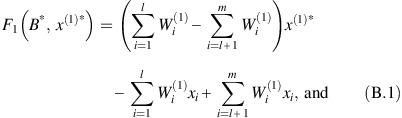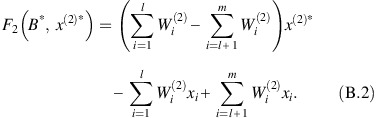Now, suppose x (1)* and x (2)* are both decreased to obtain two new x-coordinates x(1) and x(2) such that x(1)=x (1)*d=x l and x(2)=x (2)*d=x l +a, where both a and d are positive. (Note that we do not decrease either y (1)* or y (2)*; ie y(1)=y (1)* and y(2)=y (2)*.)

Then, we get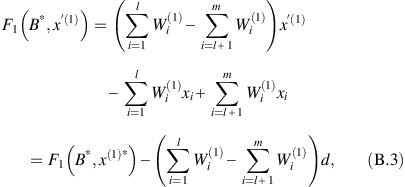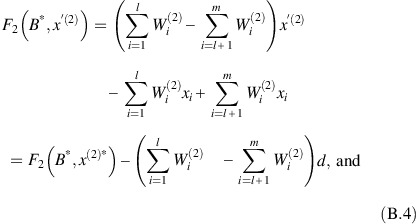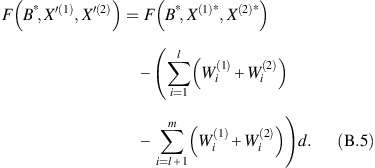Noting that W i (1)+W i (2)=∫0 B* w i (t)dt+∫ B* T w i (t)dt=∫0 T w i (t)=V i (T) and the above equations, we obtainNow, suppose that both y (1)* and y (2)* are increased to obtain two new x-coordinates x(1) and x(2) such that x(1)=x (1)*+b=x l+1a and x(2)=x (2)*+b=x l+1. Then, we have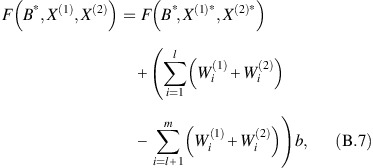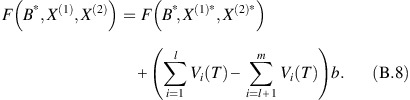However, without loss of generality, the expression ∑ i=1 l V i (T)−∑ i=l+1 m V i (T) can be equal to zero, positive, or negative. (If the expression is equal to zero, ie ∑ i=1 l V i (T)−∑ i=l+1 m V i (T), then decreasing or increasing x (1)* and x (2)*simultaneously will not change the optimal cost and hence we have multiple optimal solutions.) If it is positive, then F(B *, X(1), X(2)) is less than F(B *, X (1)*, X (2)*). If the expression is negative, then F(B *, X(1), X(2)) is less than F(B *, X (1)*, X (2)*). Therefore, there are situations in which F(B *, X(1), X(2)) or F(B *, X(1), X(2)) is less than F(B *, X (1)*, X (2)*), which implies that there is a solution that is better than the optimal solution! This is a contradiction. Therefore, an optimal solution cannot satisfy x l <x (1)*<x (2)*<x l+1 and x l <x (2)*<x (1)*<x l+1. Similarly, the optimal solution cannot satisfy y k <y (1)*<y (2)*<y k+1 and y k <y (2)*<y (1)*<y k+1. Therefore, (8) and (9) are held at an optimal solution of F. Because (8) and (9) are held at optimality, the triangle inequalities (4) and (5) will become the equalities and hence, F(A *)=H(A *). □

### Appendix C

Note that the performance of the above algorithm can be accelerated by checking if a certain BASELP has already been solved.

## Rights and permissions

Reprints and Permissions

Zanjirani Farahani, R., Szeto, W. & Ghadimi, S. The single facility location problem with time-dependent weights and relocation cost over a continuous time horizon. J Oper Res Soc 66, 265–277 (2015). https://doi.org/10.1057/jors.2013.169